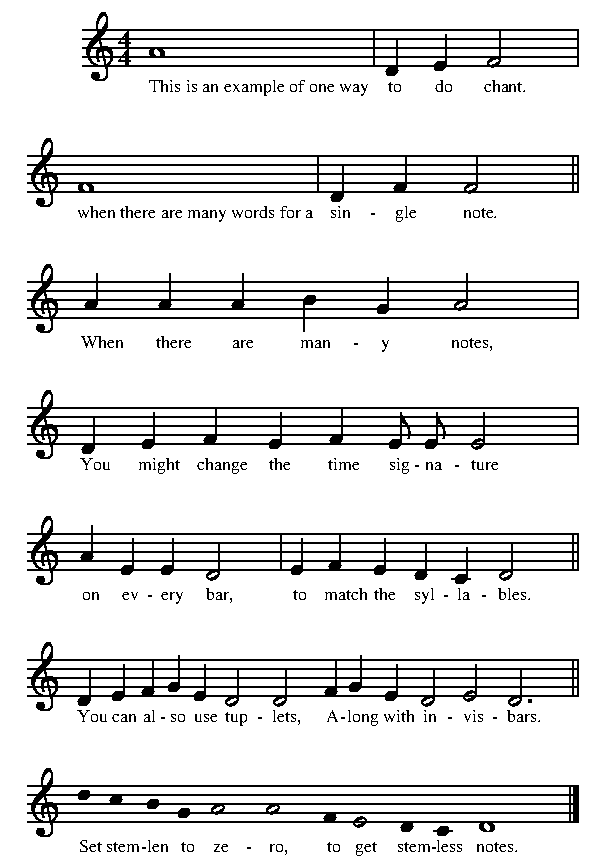## Cross-bar beaming

Generally, beams do not cross bar lines, and Mup follows that rule. However, if you want beams to cross a bar line, there are at least two possible approaches to getting the desired effect. The first is to explicitly specify stem lengths such that all the beams in the various measures will lie on the same line. You can then use the line command to fill in the gaps in the beams across the bar lines.

The other approach is to draw in the bar line using the "line" construct. To do this you first tell Mup that a measure is twice as long as is really is, so that you can put two actual measures inside what Mup thinks is a single measure. Here is an example.

```// First make an empty measure ending with an
// invisible bar. This is for the sole purpose
// of allowing the real time signature to be
// printed and would not be necessary if we
// wanted to cross a bar line other than
// the very first bar line of the piece.
1: ms;
invisbar

// Now, make the effective time signature twice
// as long as the real time signature, but use "n" so this fake
// time signature is not actually printed.
// Set up for beaming across the entire double-length measure.
// (You could could use other beamstyles if you wish, or custom beaming.)
score time=8/4n
beamstyle=1/2
music

// Now do the double-length measure. On the chord
// just after where we want a bar line, add some extra
// padding and set a location tag so that we can draw
// a bar line relative to the tag.
// Also set a location tag on the next bar line,
// so that we can get the vertical endpoints of the
// bar line that we draw from the endpoints of the
// normal Mup-supplied bar line.
bar =b

// Now draw the bar line that goes through the beam.
// Use the horizontal position relative to the "a"
// location tag on the chord in the second actual measure,
// and get the vertical endpoints from the "b" tag
// associated with the next bar line.
line (a.w + 1, b.y + 4) to (a.w + 1, b.y - 4)
```